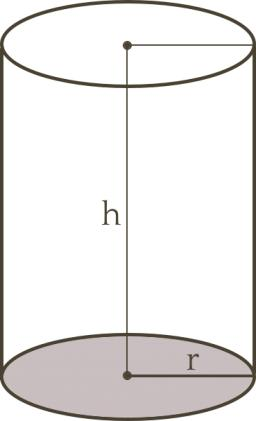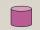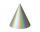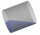# Cylinder surface, volume

The area of the cylinder surface and the cylinder jacket are in the ratio 3: 5. The height of the cylinder is 5 cm shorter than the radius of the base. Calculate surface area and volume of the cylinder.

Correct result:

S =  10367.26 cm2
V =  70685.83 cm3

#### Solution:

$\dfrac{ S_1 }{ S_2 } = \dfrac35 \ \\ \dfrac{ \pi r^2 }{ 2 \pi r h } = \dfrac35 \ \\ \dfrac{ r }{ 2 h } = \dfrac35 \ \\ 5 r = 6 h \ \\ h = r-5 \ \\ \ \\ h = 25 \ cm \ \\ r = 30 \ cm \ \\ S = 2 \pi r^2 + 2 \pi r h = 2\pi\cdot 30\cdot (30+25) = 10367.26 \ \text{cm}^2 \ \\$We would be pleased if you find an error in the word problem, spelling mistakes, or inaccuracies and send it to us. Thank you!Tips to related online calculators
Check out our ratio calculator.
Tip: Our volume units converter will help you with the conversion of volume units.

#### You need to know the following knowledge to solve this word math problem:

We encourage you to watch this tutorial video on this math problem:

## Next similar math problems:

• SequenceFind the common ratio of the sequence -3, -1.5, -0.75, -0.375, -0.1875. Ratio write as decimal number rounded to tenth.
• KitchenKitchen roller has a diameter 70 mm and width of 359 mm. How many square millimeters roll on one turn?
• Gasoline canisters35 liters of gasoline is to be divided into 4 canisters so that in the third canister will have 5 liters less than in the first canister, the fourth canister 10 liters more than the third canister and the second canister half of that in the first canister
• Common cylinderI've quite common example of a rotary cylinder. Known: S1 = 1 m2, r = 0.1 m Calculate : v =? V =? You can verify the results?
• Cylinder diameterThe surface of the cylinder is 149 cm2. The cylinder height is 6 cm. What is the diameter of this cylinder?
• Rotary cylinder 2Base circumference of the rotary cylinder has same length as its height. What is the surface area of cylinder if its volume is 250 dm3?
• Cylinder - areaThe diameter of the cylinder is one-third the length of the height of the cylinder. Calculate the surface of cylinder if its volume is 2 m3.
• Cylinder and its circumferenceIf the height of a cylinder is 4 times its circumference c, what is the volume of the cylinder in terms of its circumference, c?
• Cylinder - A&VThe cylinder has a volume 1287. The base has a radius 10. What is the area of surface of the cylinder?
• Cross-sections of a coneCone with base radius 16 cm and height 11 cm divide by parallel planes to base into three bodies. The planes divide the height of the cone into three equal parts. Determine the volume ratio of the maximum and minimum of the resulting body.
• RatioThe radii of two cones are in the ratio 5.7 Calculate the area ratio if cones have same height.
• Surface of the cylinderCalculate the surface area of the cylinder when its volume is 45 l and the perimeter of base is three times of the height.
• Surface and volumeCalculate the surface and volume of a cylinder whose height is 8 dm and the radius of the base circle is 2 dm
• JarFrom the cylinder shaped jar after tilting spilled water so that the bottom of the jar reaches the water level accurately into half of the base. Height of jar h = 7 cm and a jar diameter D is 12 cm. How to calculate how much water remains in the jar?
• Volume and surfaceCalculate the volume and surface area of the cylinder when the cylinder height and base diameter is in a ratio of 3:4 and the area of the cylinder jacket is 24 dm2.
• Cylindrical containerAn open-topped cylindrical container has a volume of V = 3140 cm3. Find the cylinder dimensions (radius of base r, height v) so that the least material is needed to form the container.
• Tin with oilTin with oil has the shape of a rotating cylinder whose height is equal to the diameter of its base. Canned surface is 1884 cm2. Calculate how many liters of oil is in the tin.